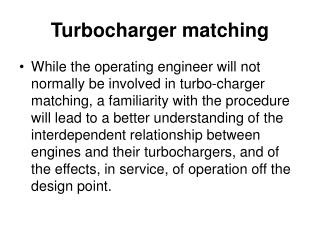DownloadDownload PresentationTurbocharger matching

# Turbocharger matching

Télécharger la présentation## Turbocharger matching

- - - - - - - - - - - - - - - - - - - - - - - - - - - E N D - - - - - - - - - - - - - - - - - - - - - - - - - - -
##### Presentation Transcript

1. Turbocharger matching • While the operating engineer will not normally be involved in turbo-charger matching, a familiarity with the procedure will lead to a better understanding of the interdependent relationship between engines and their turbochargers, and of the effects, in service, of operation off the design point.

2. Inlet conditions of the compressor P1 (after pressure drop across air suction filter) and T1 are selected. • An estimate is made of the power of the engine at a particular engine RPM. Also, an estimate is made of amount of air, ma, the engine would require at above conditions. ma = Vsw x a x vol x N (for two stroke diesel engine). The pressure in the air manifold and temperature are estimated to get the a.

3. 3. A drop across air cooler is assumed and added to air manifold pressure to get the compressor discharge pressure P2. The compressor pressure ratio P2/P1 can now be calculated. 4. The compressor frame size and its diffuser can now be selected by entering the family of compressor maps with the values of P2/P1 and Va or (maT0/P0). The operating point must have adequate margin from surge limit i.e. it must be 10% to 20% to the right of the surge limit at the value of P2/P1.

4. 5. From the performance map of the selected compressor and diffuser, the compressor efficiency (c) and turbocharger RPM are read at the operating point. 6. The required power to drive the compressor is given by Wc=maCpaT1[(P2/P1)(-1)/ -1]/ c The required turbine power Wt = Wc /mech where mech is the known characteristic of the bearings.

5. 7. Again an estimate is made of the gas conditions at the turbine inlet i.e. P3 and T3 from basic principles and empirical data including previous performance. 8. The turbine outlet pressure P4 is also estimated by adding an amount to atmospheric pressure to allow for typical losses through exhaust gas economiser. Then expansion ratio P3/P4 is calculated. 9. The mass flow rate of gas, mg, is calculated by adding mass flow rate of fuel, mf, to mass flow rate of air, ma, which was estimated previously.

6. 10. In general, the selection of a compressor wheel diameter predetermines turbine characteristics, which includes wheel diameter and blade length. With values of P3/P4 and Vg, a turbine blade and nozzle angle selection curve, can be entered for the frame size under consideration, to select nozzle opening and blade angle. 11. The following are calculated: Mean tangential velocity of blade, Um =  x Dm x RPM where Dm = mean diameter of turbine wheel Ideal gas speed at nozzle exit Cg = [2Cpg T3 {1- (P4/P3)-1/]

7. 12. The turbine efficiency  t can then be obtained from figure. • The available power of the turbine Wt = mgCpgT3 [1–(P4/P3)(-1)/] t 14. A comparison is made between the turbine power available and turbine power required. If available power is greater than required power, then the estimate of air manifold pressure, made in step 2, can be raised and the procedure is repeated with this new assumption. If the available power is lower, a lower value of air manifold pressure is assumed.

8. This iterative process is continued till the required turbine output matches the achieved value within a percent or two, with the final matching to be done by actually running the turbocharger and engine on a test-bed and making final adjustments by changing compressor diffuser vanes and turbine nozzle rings.